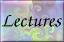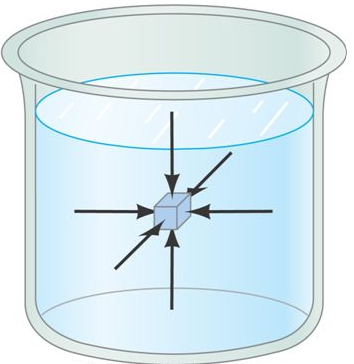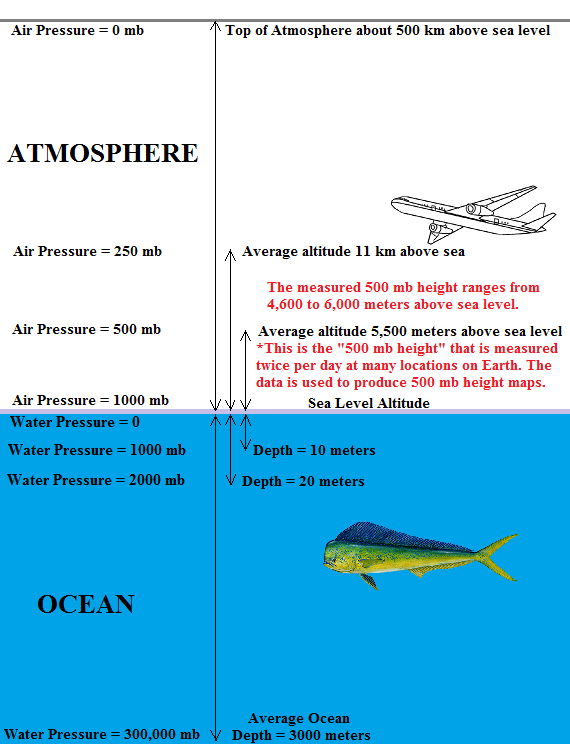# Fluid Pressure and 500 mb Height

Fluids are substances that can flow. The shape of a fluid can be easily changed, and fluids conform to the shape of their containers. Liquids and gases are fluids. Solid objects are not fluid. Fluids exert a pressure on any object immersed in the fluid. The force exerted by fluid pressure pushes inward and perpendicularly to the surface of the object. The figure below shows this for a small cubical object placed in a beaker of water. The inward force on each face is the fluid pressure multiplied by the surface area. Thus, fluid pressure is a force per area. The same principle applies for air pressure. We are immersed in air. Air pressure causes a force that pushes inward over the entire surface of our bodies.The strength of the pressure at any point in a fluid is mostly determined by the depth in the fluid. Liquid water will be discussed first. Consider diving down into a body of water. The fluid (water) pressure increases as you move vertically downward into fluid (water). This is because fluid pressure is caused by the weight per area of the fluid (water) above you. Basically, the weight per area of the overlying fluid (water) exerts a downward pressure that gets distributed in all directions by the underlying fluid. Thus, for the oceans, the water pressure is zero at the top of the ocean surface and increases in strength as you move downward. The greatest water pressure is found at the bottom of the deepest ocean.

The same principle holds for air pressure in the atmosphere. Since air pressure is determined by the weight per area of the air above a given point, the greatest air pressure is found at the bottom of the atmosphere, which is just above the ocean or ground surface. Air pressure decreases in value as you move upward and becomes zero at the top of the atmosphere because there is no weight of air above the top of the atmosphere. The general variation of air and water pressure is shown in the figure below.The average air pressure at the altitude of sea level is about 1000 mb, where mb stands for millibars, which is the unit we will use for air pressure. (When reading this material, say millibars when you encounter 'mb'). This is an average. The sea level air pressure measured at a particular location and time on Earth will typically range from about 950 mb to 1050 mb. A good approximation to remember is that the average air pressure at an altitude of sea level is about 1000 mb. Because air pressure is determined by the weight of air above a given point, the maximum air pressure above a location on the Earth occurs just above the ground, since the weight of the entire atmosphere is above. The air pressure must decrease as you move upward since there is a decreasing weight of air above you. At the top of the atmosphere, the air pressure is zero since there is no air above the top of the atmosphere.

Moving upward from sea level, the air pressure falls from about 1000 mb to zero. Thus, at some altitude above sea level, the air pressure will be 500 mb. The altitude where the measured air pressure is 500 mb is known as the 500 mb height as indicated in the figure above. The 500 mb height above a point on the Earth's surface is related to the air temperature between the ground the 500 mb height level, such that the warmer the air, the higher the 500 mb height level above the ground. Because the air temperature changes, the measured 500 mb height changes with location and time. The figure indicates that an average 500 mb height is about 5500 meters above sea level, though the 500 mb heights can range from 4600 meters to 6000 meters. Contour maps of the 500 mb height pattern at a specific time are constructed using simultaneous measurements of the 500 mb height from many locations across the surface of the Earth. We are going to use 500 mb height maps to visualize and study large scale weather patterns. The main purpose of this introduction was to describe what is meant by the 500 mb height.

The important points from this page are:
• The average air pressure at bottom of the atmosphere (just above the ground) is about 1000 mb (millibars)
• Air pressure decreases in value as you move upward, since it is determined by the weight of the remaining air above you
• The 500 mb height is the altitude (measured in meters above sea level) where the measured air pressure is 500 mb (millibars)
• Contour maps of 500 mb height are constructed based on simultaneous measurements of the 500 mb height taken around the world
• 500 mb height maps are a good way to visualize the large-scale weather pattern, which we will study next

The remaining paragraphs describe a couple of differences between air and water with respect to vertical changes in fluid pressure. You may wish to skip this for now. These ideas will be covered again in later reading assignments.

In both liquid water and air, the fluid pressure at any point is determined by the weight per area of the fluid above that point. However, there are important differences between air and liquid water. Liquid water is much denser than air, which simply means that an equal volume filled with liquid water weighs much more than one filled with air. This means that the water pressure at the bottom of the ocean is much larger than the air pressure at the bottom of the atmosphere, even though the entire atmosphere is taller than the depth of the ocean. In fact, the water pressure at a depth of 10 meters (32 feet) is about the same as the average air pressure at sea level. This means that the weight per area of a 10 meter tall column of water is the same as the weight per area of the entire column of air in the Earth's atmosphere, from sea level to the top of the atmosphere, which is around 500 kilometers above sea level. The average sea level air pressure is often termed one atmosphere of pressure.

Liquid water is an incompressible fluid, which means a given volume of water cannot be squeezed together to become denser. Water at the bottom of the ocean is approximately the same density as water at the top of the ocean since liquid water is not compressed by the enormous weight of the water piled above it. Because liquid water density does not change with depth, water pressure increases at a constant rate with increasing depth. From the previous paragraph, this means that water pressure increases by about one atmosphere (approximately 1000 mb) for every 10 meter increase in depth. The increase in water pressure with depth limits how deep scuba divers and submarines can dive. The average depth of the ocean is about 3000 meters. The water pressure at this depth is 300 times larger than the average sea level air pressure. Even larger water pressures happen at the bottom of deep ocean trenches.

The air in the atmosphere is in the form of a gas, rather than a liquid. Gases are compressible fluids, which means a given volume of air can be easily squeezed together to become denser. Air at the bottom of the atmosphere is compressed (squeezed together) by the weight of the air piled above it. The most dense air is at the bottom of the atmosphere (since that air is being compressed by the weight of the entire atmosphere above it), and the air density decreases at higher altitudes (since a decreasing amount and weight of atmosphere is above higher altitudes). Because air density does change with height above the ground surface, air pressure does not decrease at a constant rate with increasing altitude as in liquid water. At the altitude where the air pressure is 500 mb, half of the total weight of the atmosphere is above that altitude and the other half is below that altitude. (The total weight of the atmosphere causes 1000 mb of air pressure, thus where the air pressure is 500 mb, there is exactly half the total weight of the atmosphere above that altitude). Basically, half of the Earth's atmosphere is compressed within about 5.5 km (5,500 meters) of the ground surface, while the other half is spread out from 5.5 km above sea level to the top of the atmosphere, which is around 500 km (500,000 meters) above sea level. The changing air density causes the air pressure to decrease exponentially with increasing altitude, rather than at a constant rate (linearly). For Earth's atmosphere, the air pressure roughly drops in half for every 5,500 meter increase in elevation. Notice in the diagram that air pressure dropped by half, from 1000 mb to 500 mb, in moving from sea level to 5,500 meters above sea level. The pressure drops by half again after moving up another 5,500 meters, from 500 mb at 5,500 meters above sea level to 250 mb at 11,000 meters (or 11 km) above sea level. Please do not be discouraged if you are having trouble following all of the concepts described in this paragraph. At this point, it is most important that you understand what is meant by the 500 mb height in the atmosphere.